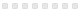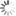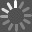# Singular Limits in Thermodynamics of Viscous Fluids

eBook - 2009
Rate this:Springer Publishing

Many interesting problems in mathematical fluid dynamics involve the behavior of solutions of nonlinear systems of partial differential equations as certain parameters vanish or become infinite. Frequently the limiting solution, provided the limit exists, satisfies a qualitatively different system of differential equations. This book is designed as an introduction to the problems involving singular limits based on the concept of weak or variational solutions. The primitive system consists of a complete system of partial differential equations describing the time evolution of the three basic state variables: the density, the velocity, and the absolute temperature associated to a fluid, which is supposed to be compressible, viscous, and heat conducting. It can be represented by the Navier-Stokes-Fourier-system that combines Newton's rheological law for the viscous stress and Fourier's law of heat conduction for the internal energy flux.

As a summary, this book studies singular limits of weak solutions to the system governing the flow of thermally conducting compressible viscous fluids.

Many problems in mathematical fluid dynamics involve the behavior of solutions of nonlinear systems of partial differential equations. This book is an introduction to the problems involving singular limits based on the concept of weak or variational solutions.

Publisher: Basel : Birkhäuser Basel, 2009
ISBN: 9783764388430
Characteristics: v.: digital
Additional Contributors:## Opinion

### From the critics### Community Activity

#### Comment

There are no comments for this title yet.

#### Age Suitability

There are no age suitabilities for this title yet.

#### Summary

There are no summaries for this title yet.

#### Notices

There are no notices for this title yet.

#### Quotes

There are no quotes for this title yet.## Explore Further### Subject HeadingsLoading...

# Find it at NPLLoading...
[]
[]